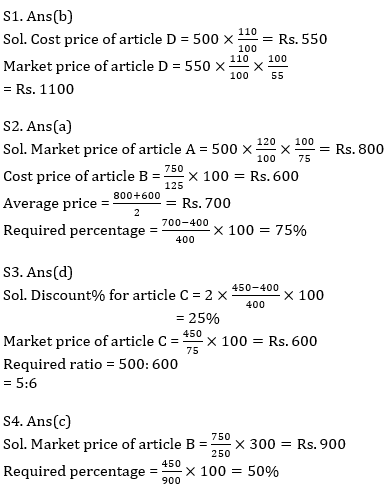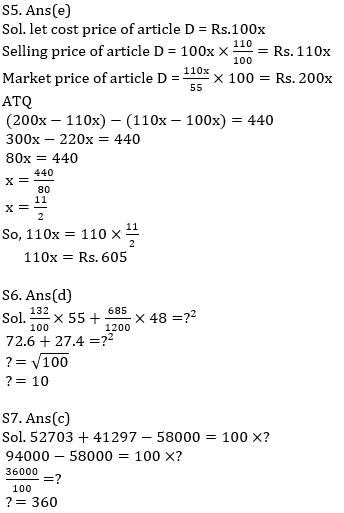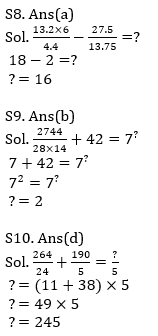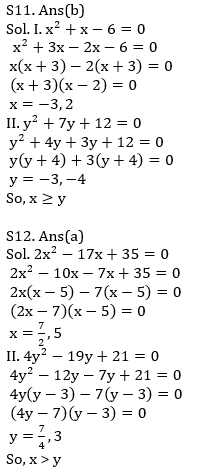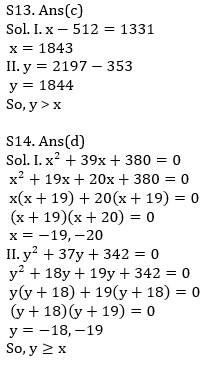Latest Banking jobs   »   RBI Assistant Vacancy 2022   »   Quantitative Aptitude Quiz For RBI Assistant...

# Quantitative Aptitude Quiz For RBI Assistant Prelims 2022- 19th March

Directions (1-5): Table given below gives information about Cost price, Selling price, profit percent and discount percentage of four article A, B, C and D. Some data is missing, calculate the data and answer the following questions.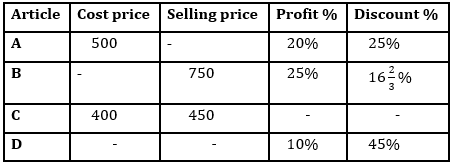Q1. If cost price of article D is 10% more than cost price of article A, find Market price of article D?
(a) Rs. 1000
(b) Rs. 1100
(c) Rs. 900
(d) Rs. 950
(e) Rs. 1050

Q2. Average of market price of article A and cost price of article B is how much percent more than cost price of article C?
(a) 75%
(b) 80%
(c) 60%
(d) 90%
(e) 95%

Q3. If discount percentage is twice of profit percentage for article C, find ratio of cost price of article A to market price of article C?
(a) 2:3
(b) 1:2
(c) 2:5
(d) 5:6
(e) None of these.

Q4. Selling price of article C is what percent of market price of article B?
(a) 100%
(b) 75%
(c) 50%
(d) 25%
(e) 60%

Q5. If for article D difference between profit earned and discount given is Rs. 440, find selling price of the D?
(a) 550
(b) 650
(c) 308
(d) 450
(e) 605

Directions (6-10): Simplify the following equations and find the value of (?) question mark?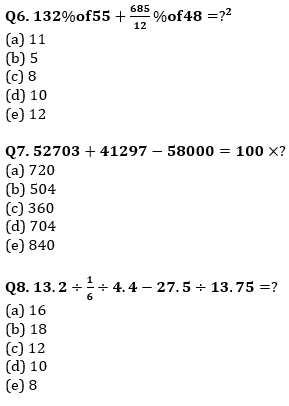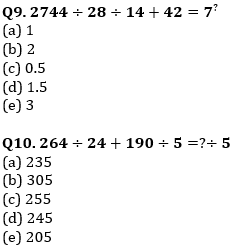Directions (11-15): In each of the following questions, two equations (I) and (II) are given, you have to solve both the equations and give answer.
(a) If x > y
(b) If x ≥ y
(c) If x < y
(d) If x ≤ y
(e) If x = y or no relation can be established between x and y.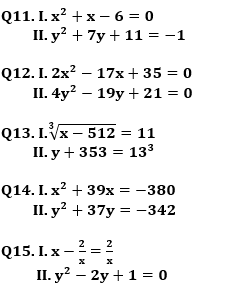Solutions